Updating search results...

# 16 Results

View
Selected filters:
• symmetry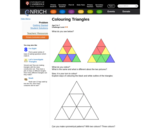Some Rights Reserved
Rating
0.0 stars

This activity allows students to explore reflective symmetry. They are asked to color a given arrangement of triangles in symmetric patterns using specific numbers of colors. The Teachers' Notes page includes suggestions for implementation, discussion questions, ideas for extension and support, and printable sheets

Subject:
Mathematics
Material Type:
Activity/Lab
Provider:
NRICH
01/05/2009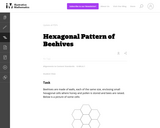Unrestricted Use
CC BY
Rating
0.0 stars

The goal of this task is to use geometry study the structure of beehives. Beehives have a tremendous simplicity as they are constructed entirely of small, equally sized walls. In order to as useful as possible for the hive, the goal should be to create the largest possible volume using the least amount of materials. In other words, the ratio of the volume of each cell to its surface area needs to be maximized. This then reduces to maximizing the ratio of the surface area of the cell shape to its perimeter.

Subject:
Mathematics
Secondary Mathematics
Material Type:
Activity/Lab
Provider:
Illustrative Mathematics
Provider Set:
Illustrative Mathematics
Author:
Illustrative Mathematics
01/21/2013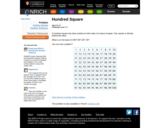Some Rights Reserved
Rating
0.0 stars

This activity provides an opportunity for students to work on spatial visualization and number sense together. Learners are presented with a hundred grid and told there is another printed on the reverse side. They must work out the unseen numbers for several positions on the grid. Ideas for implementation, extension and support are included along with a printable sheet of grids.

Subject:
Mathematics
Material Type:
Activity/Lab
Provider:
NRICH
10/05/2009Some Rights Reserved
Rating
0.0 stars

This activity allows students to investigate line symmetry and reflections. Using a mirror, students locate the lines of symmetry. in a square and then proceed to find other shapes by reflecting parts of the square. Ideas for implementation, extension and support are included along with a printable worksheet of squares (.doc)

Subject:
Mathematics
Material Type:
Activity/Lab
Provider:
NRICH
08/05/2003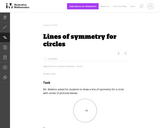Unrestricted Use
CC BY
Rating
0.0 stars

This is an instructional task that gives students a chance to reason about lines of symmetry and discover that a circle has an an infinite number of lines of symmetry. Even though the concept of an infinite number of lines is fairly abstract, fourth graders can understand infinity in an informal way.

Subject:
Elementary Mathematics
Mathematics
Material Type:
Activity/Lab
Provider:
Illustrative Mathematics
Provider Set:
Illustrative Mathematics
Author:
Illustrative Mathematics
11/11/2012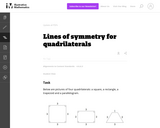Unrestricted Use
CC BY
Rating
0.0 stars

This task provides students a chance to experiment with reflections of the plane and their impact on specific types of quadrilaterals. It is both interesting and important that these types of quadrilaterals can be distinguished by their lines of symmetry.

Subject:
Elementary Mathematics
Mathematics
Material Type:
Activity/Lab
Provider:
Illustrative Mathematics
Provider Set:
Illustrative Mathematics
Author:
Illustrative Mathematics
11/11/2012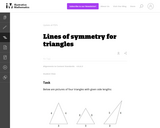Unrestricted Use
CC BY
Rating
0.0 stars

This task is intended for instruction, providing the students with a chance to experiment with physical models of triangles, gaining spatial intuition by executing reflections.

Subject:
Elementary Mathematics
Mathematics
Material Type:
Activity/Lab
Provider:
Illustrative Mathematics
Provider Set:
Illustrative Mathematics
Author:
Illustrative Mathematics
10/12/2012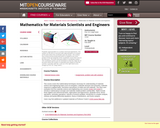Conditional Remix & Share Permitted
CC BY-NC-SA
Rating
0.0 stars

This course covers the mathematical techniques necessary for understanding of materials science and engineering topics such as energetics, materials structure and symmetry, materials response to applied fields, mechanics and physics of solids and soft materials. The class uses examples from the materials science and engineering core courses (3.012 and 3.014) to introduce mathematical concepts and materials-related problem solving skills. Topics include linear algebra and orthonormal basis, eigenvalues and eigenvectors, quadratic forms, tensor operations, symmetry operations, calculus of several variables, introduction to complex analysis, ordinary and partial differential equations, theory of distributions, and fourier analysis. Users may find additional or updated materials at Professor Carter's 3.016 course Web site.

Subject:
Mathematics
Material Type:
Full Course
Provider:
M.I.T.
Provider Set:
M.I.T. OpenCourseWare
Author:
Carter, W. Craig
01/01/2005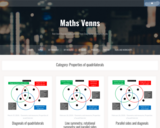Restricted Use
Rating
0.0 stars

How well do your students understand the properties of quadrilaterals and the vocabulary that is used to describe them? Use these Venn diagrams as a way for students to prove which quadrilateral is being described.

Subject:
Mathematics
Material Type:
Activity/Lab
Assessment
Homework/Assignment
Provider:
Maths Venn
Author:
Craig Barton
10/24/2022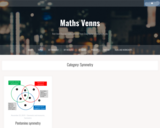Restricted Use
Rating
0.0 stars

Use this Venn diagram to get students thinking about the different types of symmetry as they use the mathematical practices.

Subject:
Mathematics
Material Type:
Activity/Lab
Assessment
Homework/Assignment
Provider:
Maths Venn
Author:
Craig Barton
10/24/2022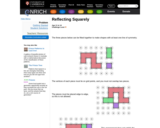Some Rights Reserved
Rating
0.0 stars

In this problem students use spatial awareness and visualization to solve problems related to reflection (bilateral) symmetry. Learners are given three shapes and must assemble as many different but symmetrical composites as possible. Ideas for implementation, extension and support are included along with printable sheets of the shapes and a poster.

Subject:
Mathematics
Material Type:
Activity/Lab
Provider:
NRICH
04/05/2009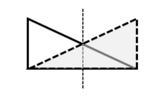Conditional Remix & Share Permitted
CC BY-NC
Rating
0.0 stars

This is a lesson plan that introduces rotational and reflectional symmetry with geometric shapes, focusing on regular polygons. Notes (two copies: a student version and also an answer key, both as a Word doc and a PDF), assignment, and cut-out shapes are provided.

Subject:
Secondary Mathematics
Material Type:
Homework/Assignment
Lesson
Author:
Kimberly
02/07/2022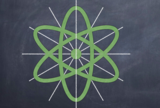Conditional Remix & Share Permitted
CC BY-NC
Rating
0.0 stars

This is a lesson plan that introduces rotational and reflectional symmetry with geometric shapes, focusing on regular polygons. Notes (two copies: a student version and also an answer key, both as a Word doc and a PDF), assignment, and cut-out shapes are provided. There is a final project, with both instructions and an example included. Cover image: Christensen, K., 2022. Reflectional Symmetry of an Atom [screenshot] (Keynote presentation, Rotational and Reflectional Symmetry Assignment).

Subject:
Secondary Mathematics
Material Type:
Activity/Lab
Assessment
Diagram/Illustration
Homework/Assignment
Lesson Plan
Author:
Kimberly
03/09/2022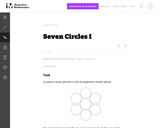Unrestricted Use
CC BY
Rating
0.0 stars

This task is intended to help model a concrete situation with geometry. Placing the seven pennies in a circular pattern is a concrete and fun experiment which leads to a genuine mathematical question: does the physical model with pennies give insight into what happens with seven circles in the plane?

Subject:
Mathematics
Secondary Mathematics
Material Type:
Activity/Lab
Provider:
Illustrative Mathematics
Provider Set:
Illustrative Mathematics
Author:
Illustrative Mathematics
08/20/2012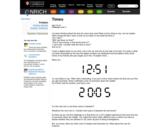Some Rights Reserved
Rating
0.0 stars

In this activity learners explore the connections of digital time displays with numeric and geometric properties. Students look for times that have bilateral or rotational symmetry, or have a certain digital sum, etc. Ideas for implementation, extension and support are included.

Subject:
Mathematics
Material Type:
Activity/Lab
Provider:
NRICH
08/05/1998Restricted Use
Rating
0.0 stars

Have you ever thought about the mathematics of beading? This challenge will have you thinking about symmetry and all of the ways you can create different necklaces with the same beads.

Subject:
Mathematics
Material Type:
Activity/Lab
Lesson
Teaching/Learning Strategy
Provider: